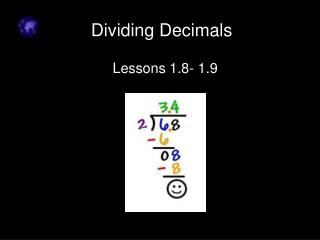DownloadDownload PresentationDividing Decimals

# Dividing Decimals

Download Presentation## Dividing Decimals

- - - - - - - - - - - - - - - - - - - - - - - - - - - E N D - - - - - - - - - - - - - - - - - - - - - - - - - - -
##### Presentation Transcript

1. Dividing Decimals Lessons 1.8- 1.9

2. Multiplying and Dividing Decimals by 10, 100, and 1,000

3. Tips: • When you multiply by 10, 100, or 1,000, you can move the decimal point to the right. • The number of decimal places you move is the same as the number of zeroes you are multiplying by. • When you divide by 10, 100, or 1,000, you can move the decimal point to the left. Move the decimal point once for each zero you are dividing by.

4. 1.492 x 100 Move the decimal to the right: two spaces. 149.2

5. 4.2 ÷ 100 Move the decimal point to the left: two spaces. Add a zero for the second space. .042

6. Dividing Decimals

7. Remember the parts of a division problem: quotient 9 5 45 dividend divisor

8. Dividing a Decimal by a Whole Number • Place the decimal point in the quotient directly above the decimal point in the dividend. • Divide as you normally would (DMSCB)

9. Example DMSCB 2 . 5 5 12.5 10 2 5 25 0

10. 1 1 . 2 8 You Try! 9 101.52 9 1 1 9 2 5 18 7 2 72 0

11. Dividing a Decimal by a Decimal • Move the decimal point in the divisor to the right, until you get to the end of the digits. • Count how many places you moved that decimal. • Move the decimal point in the dividend the same numberof places to the right that you moved the decimal point in the divisor.

12. Example 0.3 6.72 one space 03 67.2 New problem

13. 2 2 . 4 DMSCB 3 67.2 6 0 7 6 1 2 1 2 0

14. Your Turn! 3.2 2.24 . 7 32 32 x 5 x 8 32 22.4 160 256 22 4 32 0 x 7 224

15. Example 3 0.38 13.3 The decimal must be moved 2 spaces to the right. When we move this one 2 spaces, we find that we need to add a space. What will we fill it with? Answer: Fill it in with a 0. 13.3 becomes 1330 SO, our problem becomes 038 into 1330. 038 is the same as 38.

16. 248 248 . 5 4 x 5 x 6 1240 1488 248 133.92 124 0 248 x 4 9 9 2 992 9 92 0

17. Terminating and Repeating Decimals • A terminating decimal is a decimal that stops, or terminates. • Examples: 1.25 or 0.892 • A repeating decimal is a decimal that has a repeating digit or a repeating group of digits. • Examples: 1.3333… or .121212121… • Repeating decimals usually have a bar across the repeating portion. 2.3

18. 5 7 . 7 62 0 8 .0 56 6 0 Terminating decimal 56 4 0 40 0

19. . 8 3 3 5 .0 0 0 6 This is a repeating decimal. 4 8 .83 2 0 18 2 0 18 2

20. Classwork:Try this Decimals Cruncher game to practice dividing decimals. Use this interactive Exploring Division of Decimals to understand the relationship between Multiplication and Division of decimals. pg. 45, #2-52 evens Time For Homework!